Project

# Use LaunchPad to Make a Laser Tripwire Alarm

November 18, 2015 by Tim Youngblood

## Use the Analog to Digital Converter (ADC) feature of LaunchPad to build a laser tripwire alarm. This is a great way to familiarize yourself with LaunchPad while simultaneously making something cool.

Build a laser tripwire alarm? Yes please!

### Understanding the Analog to Digital Conversion in Launchpad

The MSP430 microcontroller of LaunchPad has a built-in 10-Bit Analog to Digital Converter (ADC) module which converts the analog voltage applied to its input into a digital number. In this article we will show you how to use the ADC of the LaunchPad and build an example application developed in Energia IDE.

• 10-Bit Resolution
• 200 ksps Conversion Rate
• Successive Approximation Register (SAR) Core
• 8 Separate Input Channels
• Programmable Internal Voltage Reference
• External Voltage Reference Input
• Programmable Sample and Hold Circuit

The 10-Bit ADC module converts the input voltage to a number between 0 and 1023, which are the lower and the upper voltage limits VR+ and VR-, respectively. The MSP430 lets the user select many different reference limits, which can be a combination of supply voltage (VCC) and ground, external reference inputs VREF+/VEREF+ and VREF-/VEREF-, and internal voltage generators 2.5V and 1.5V. Assuming we use the supply voltage VCC (3.6V) and GND as the reference limits, the readings 0 and 1023 will represent 0 and 3.6V respectively and any ADC reading between them can be calculated by using the equation below.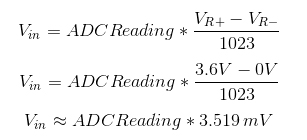In Energia, the ADC reference source can be selected by using the analogReference(option) function. The options of this function are DEFAULT (VR+ = VCC, VR- = 0V), INTERNAL1V5 (VR+ = 1.5V, VR- = 0V), INTERNAL2V5 (VR+ = 2.5V, VR- = 0V), EXTERNAL (VR+ = VREF, VR- = 0V). More options can be achieved by direct accessing the ADC10CTL0 register.

The voltage applied to an analog input pin must be within the voltage range VR- to VR+ to get a valid conversion result. The absolute maximum voltage rating of the analog input pins is VCC.

There are eight analog input pins of the LaunchPad from A0 to A7 which are shown on the image below. Unfortunately they are not marked on the LaunchPad PCB.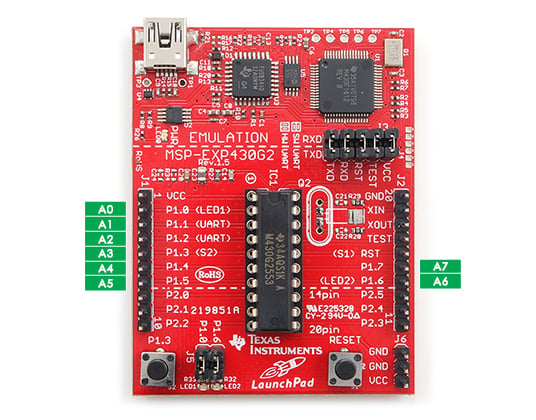In Energia, analogRead() function is used to read the analog voltage applied to a selected channel and return an integer from 0 to 1023. This function requires the channel number as the input. A hardware multiplexer connects the selected channel to the ADC module internally while the rest of the channels are kept isolated. For example “adcreading = analogRead(A4);“ command reads the analog voltage applied to the pin P1.4 (A4) and writes the result to the int type variable “adcreading.” Each conversion takes about 100 microseconds.

During the analog to digital conversion, noise on the analog signal may distort the conversion result and cause erroneous readings. To increase the signal to noise ratio (SNR), using the oversampling and averaging technique is a good practice. For example, reading five samples successively and taking their average will give more accurate result instead of reading a single sample.

There are some other channels internally connected to the ADC module (see ADC10CTL1 register for more information). One of them is the output of the internal temperature sensor. To read the temperature sensor data, just use analogRead(TEMPSENSOR); command.

### Laser Tripwire Alarm Project

It's time to build a sample application using the ADC feature of LaunchPad. We will use a laser diode, a light dependent resistor, a buzzer, a resistor, and LaunchPad to build a laser tripwire alarm.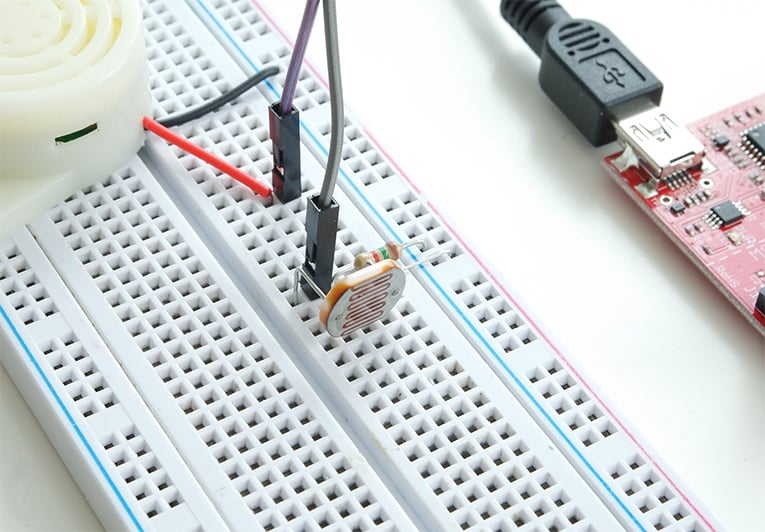A llight dependent resistor (LDR) is a semiconductor whose resistor changes depending on the light intensity on its surface. In a dark environment its resistance can increase up to mega-ohms, and in the light its resistance decreases down to a few hundred ohms. The characteristic curve of an LDR is given below.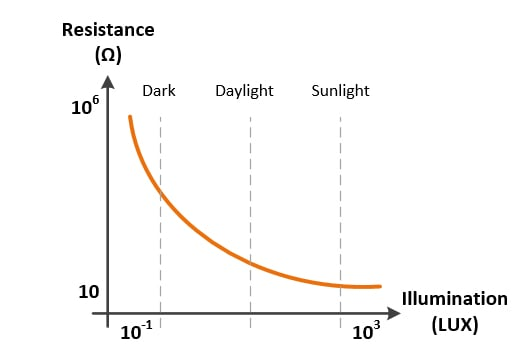The LDR is used as the laser sensor in our application. In a room with daylight, the resistance of the LDR is about 1-2 kilo-ohms. When the laser beam falls on the LDR surface, its resistance decreases down to 100-200 ohms. We need to know the resistance of the LDR to detect whether the tripwire is cut. Since the ADC module of the LaunchPad can read analog voltage, we should convert the resistance information to voltage. To achieve this, a series resistance is connected to the LDR and the VCC voltage is applied to this resistor network. Depending on the LDR resistance, the voltage output of this divider changes. You can see the schematic of the application below.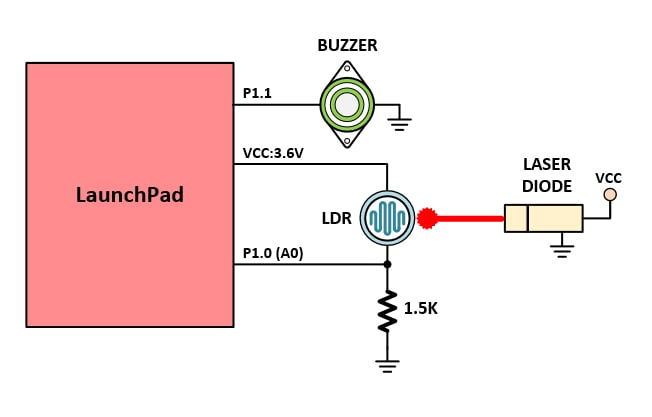When the laser beam falls on the LDR surface, the resistance of the LDR is measured about 200 ohms. At this condition, the voltage on the A0 input becomes [3.6V/(1.5K + 0.2K)]  * 1.5K = 3.18V.  When the tripwire is cut, the resistance of the LDR will increase and the voltage on the A0 input will decrease. So we can say that if we read less than 3.0V from A0 input, the wire is cut and the alarm should be activated.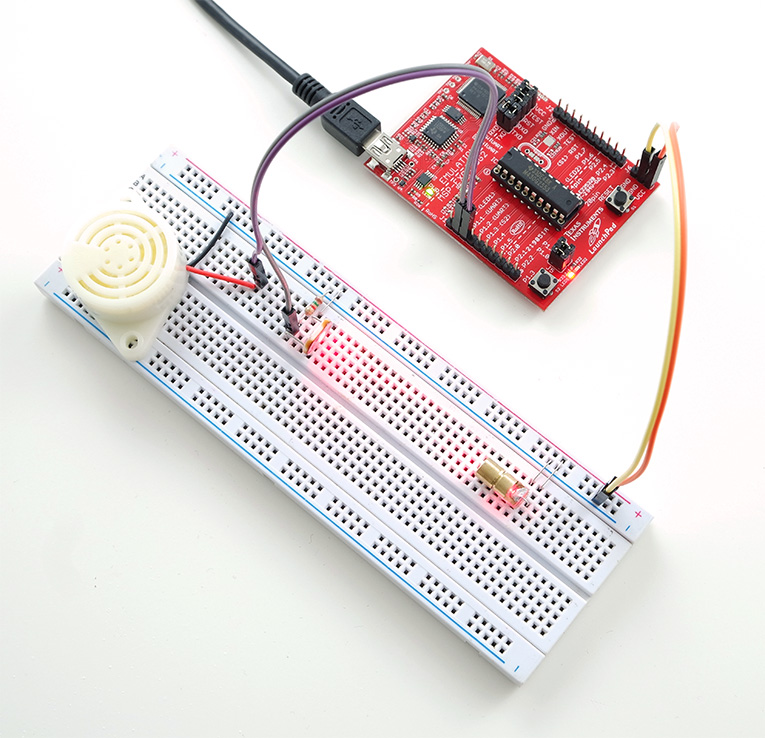The Energia sketch of the application is given below.

### Code

// the setup routine runs once when you press reset:
void setup() {
analogRefrence(DEFAULT); // Set VR+ = VCC:3.6B, VR- = GND:0V as the upper and the lower limits
pinMode(3,OUTPUT); // set the buzzer pin mode
}

// the loop routine runs over and over again forever:
void loop() {

// read the analog voltage at A0
float voltage = sensorValue * (3.6 / 1023);

if (voltage < 3.0) {

// tripwire is cut: activate the buzzer with oscillation
digitalWrite(3,HIGH);
delay(150);
digitalWrite(3,LOW);
delay(100);
}

else {
// tripwire is not cut: de-activate the buzzer
digitalWrite(3,LOW);
}

}


Laser_Tripwire.zip

You can see the laser tripwire alarm in action in the following video:

### Video

Give this project a try for yourself! Get the BOM.• S
supergravity December 08, 2015

Neat project, the laser trip wire could be used in a magnetic levitator that I made years ago, the beam would turn an electro -magnet on and off when the object breaks the beam.I was able to levitate small metal pieces about two inches beleow the coil. It had some oscillation at first but after a positive feedback resistor added to the circuit it was smooth and made quit the conversation piece.

Like.
• C
cuyler1 February 19, 2016
i would love to see the schematic for what you have built (mag lev).or can you direct me to a site. thanks cuyler
Like.
•Rayregula June 08, 2016

Could you convert it for use with an Arduino uno without to much hassle?

Like.